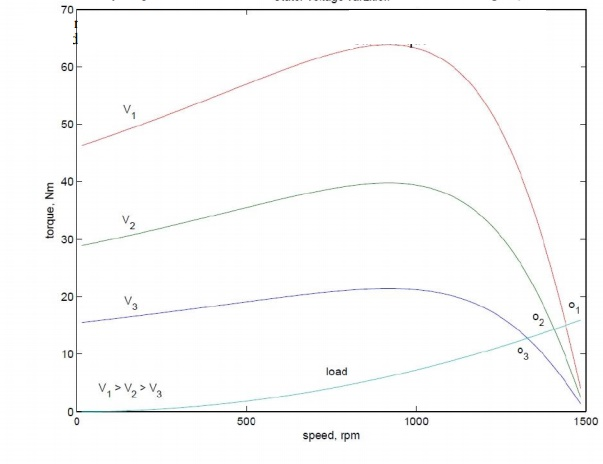Home | | Electrical Machines II | Changing applied voltage - Speed control of Three Phase Induction Motors

# Changing applied voltage - Speed control of Three Phase Induction Motors

From the torque equation of the induction machine we can see that the torque depends on the square of the applied voltage.

Speed control by changing applied voltage

From the torque equation of the induction machine we can see that the torque depends on the square of the applied voltage. The variation of speed torque curves with respect to the applied voltage is shown in Fig:. These curves show that the slip at maximum torque remains same, while the value of stall torque comes down with decrease in applied voltage. The speed range for stable operation remains the same.

Further, we also note that the starting torque is also lower at lower voltages. Thus, even if a given voltage level is sufficient for achieving the running torque, the machine may not start. This method of trying to control the speed is best suited for loads that require very little starting torque, but their torque requirement may increase with speed.

Fig: 3.28 also shows a load torque characteristic — one that is typical of a fan type of load. In a fan (blower) type of load, the variation of torque with speed is such that T ω2. Here one can see that it may be possible to run the motor to lower speeds within the range  ns to (1 ˆs) ns. Further, since the load torque at zero speed is zero, the machine can start even at reduced voltages. This will not be possible with constant torque type of loads.One may note that if the applied voltage is reduced, the voltage across the magnetising branch also comes down. This in turn means that the magnetizing current and hence flux level are reduced. Reduction in the flux level in the machine impairs torque production which is primarily the explanation for Fig: 3.28. If, however, the machine is running under lightly loaded conditions, then operating under rated flux levels is not required. Under such conditions, reduction in magnetizing current improves the power factor of operation. Some amount of energy saving may also be achieved.

Voltage control may be achieved by adding series resistors (a lossy, inefficient proposition), or a series inductor / autotransformer (a bulky solution) or a more modern solution using semiconductor devices. A typical solid state circuit used for this purpose is the AC voltage controller or AC chopper.

Study Material, Lecturing Notes, Assignment, Reference, Wiki description explanation, brief detail
Electrical machines : Starting and Speed Control of Three Phase Induction Motor : Changing applied voltage - Speed control of Three Phase Induction Motors |